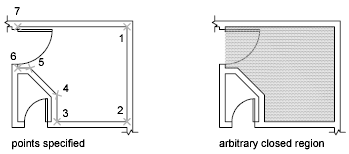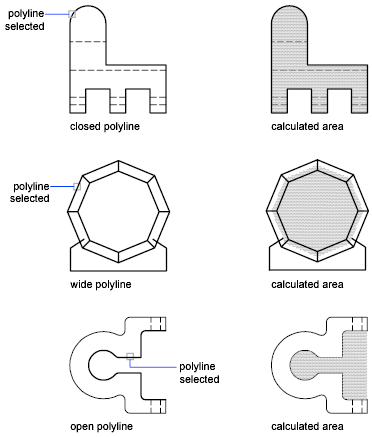# About Finding Area and Mass Properties Information

You can obtain the area, perimeter, and mass properties of selected objects or from a sequence of points.

## Use Commands to Calculate Area

With the MEASUREGEOM and AREA commands, you can specify a series of points or select an object to find the enclosed area.

In addition to area, with the MEASUREGEOM command, you can obtain geometric information from objects such as distance, radius, angle, and volume.

Tip: A fast way to find the area bounded by several objects in 2D is to use the BOUNDARY command to create a closed region or polyline. You can then use the Properties palette or the LIST command to find the area and perimeter.

## Define an Area

You can measure an area enclosed by the points you specify. The points must lie on a plane parallel to the XY plane of the current UCS.## Calculate the Area, Perimeter, or Circumference of an Object

You can calculate the enclosed area and perimeter or circumference of circles, ellipses, polylines, polygons, regions, and 3D solids. The information displayed depends on the type of object selected:

• Circles. Displays area and circumference information.
• Ellipses, closed polylines, polygons, planar closed spline curves, and regions. Displays area and perimeter information. For wide polylines, this area is defined by the center of the width.
• Open objects such as open spline curves and open polylines. Displays the area and length; the area is calculated as though a straight segment connects the start and end.
• AutoCAD 3D solids. Displays the total 3D area of the object.

## Example: How Various Areas Are Calculated## Calculate Combined Areas

You can calculate the total area of multiple areas by specifying points or by selecting objects. For example, you can measure the total area of selected rooms in a floor plan.

You can subtract areas from other areas. more than one area from a combined area as you calculate. For example, if you have calculated the area of a floor plan, you can subtract the area of a room.

If you convert enclosed areas into regions, you can add or subtract them to create a composite area, and use the Properties palette or LIST command to find the information you need.

## Calculate Mass Properties

With the MASSPROP command, you can analyze 2D regions and 3D solids for their mass properties including volume, area, moments of inertia, center of gravity, and so on. The result of the computations can be saved to a text file.